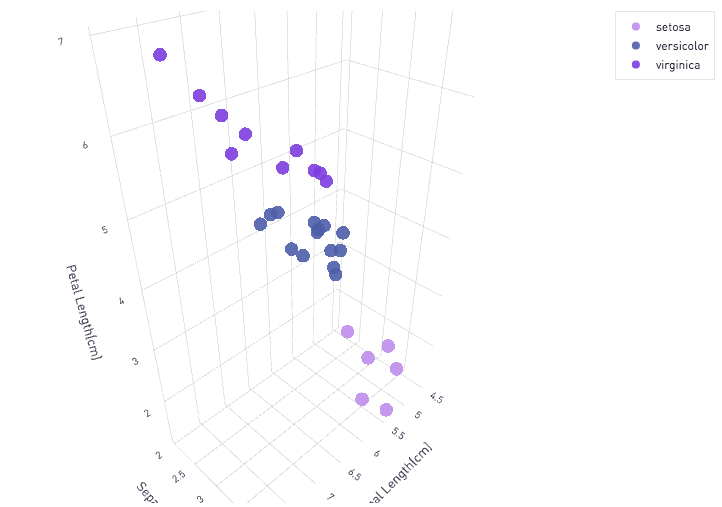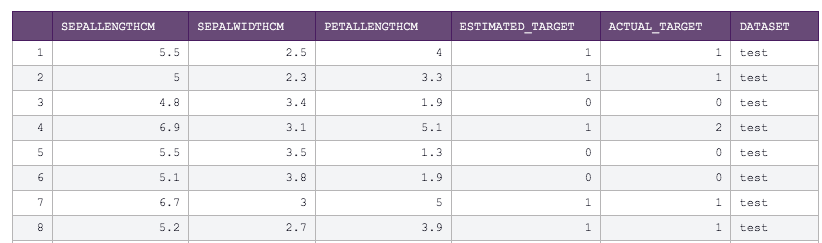# 3D Scatter plots in Plot.lyNOTE: you don't need to be doing Machine Learning to make a cool 3D plot like this! This post takes the output of a machine learning model and shows the results on a 3D plot, but you can plot almost anything with 3 numerical dimensions using basically the same script below.

For those of your who are following along on my posts on using K-Nearest-Neighbors to train a model on the Iris Dataset in Periscope (scroll down for links to these posts), I thought it would be fun to visualize my test data in Periscope on a 3D plot!

Note, your machine learning model likely will be trained on more than 3 features. However, I thought this was nifty to demonstrate spatially which labels are assigned to our testing data

This was my SQL code to grab all of my test data (to see how I built the knn_model view, check out the post here!)

`select * from [knn_model] where dataset = 'test'`

Below are the first few rows of the SQL output:And here's the Python 3.6 code, using the plot.ly library

```# SQL output is imported as a dataframe variable called 'df'
import pandas as pd
import plotly.plotly as py
import plotly.graph_objs as go

# 3D scatter plot. Resource: https://plot.ly/python/3d-scatter-plots/

setosa = go.Scatter3d(
x = df.where(df['ESTIMATED_TARGET'].astype(int) == 0)['SEPALLENGTHCM'],
y = df.where(df['ESTIMATED_TARGET'].astype(int) == 0)['SEPALWIDTHCM'],
z = df.where(df['ESTIMATED_TARGET'].astype(int) == 0)['PETALLENGTHCM'],
mode ='markers',
name = 'setosa',
marker =dict(
color = 'rgb(198, 151, 237)',
size = 8,
opacity = 0.9
)
)

versicolor  = go.Scatter3d(
x= df.where(df['ESTIMATED_TARGET'].astype(int) == 1)['SEPALLENGTHCM'],
y= df.where(df['ESTIMATED_TARGET'].astype(int) == 1)['SEPALWIDTHCM'],
z= df.where(df['ESTIMATED_TARGET'].astype(int) == 1)['PETALLENGTHCM'],
mode='markers',
name = 'versicolor',
marker=dict(
color='rgb(87, 104, 178)',
size=8,
symbol='circle',
opacity=0.9
)
)

virginica  = go.Scatter3d(
x = df.where(df['ESTIMATED_TARGET'].astype(int) == 2)['SEPALLENGTHCM'],
y = df.where(df['ESTIMATED_TARGET'].astype(int) == 2)['SEPALWIDTHCM'],
z = df.where(df['ESTIMATED_TARGET'].astype(int) == 2)['PETALLENGTHCM'],
mode = 'markers',
name = 'virginica',
marker = dict(
color = 'rgb(136, 68, 226)',
size = 8,
symbol = 'circle',
opacity = 0.9
)
)

data = [setosa , versicolor , virginica ]
layout = go.Layout(
scene = dict(xaxis = dict(title='Sepal Length(cm)'),
yaxis = dict(title='Sepal Width(cm)'),
zaxis = dict(title='Petal Length(cm)'),),
margin=dict(
l=10,
r=10,
b=10,
t=10
)
)
fig = go.Figure(data=data, layout=layout)

# Use Periscope to visualize a dataframe, text, or an image by passing data to periscope.table(), periscope.text(), or periscope.image() respectively.
periscope.plotly(fig)
```